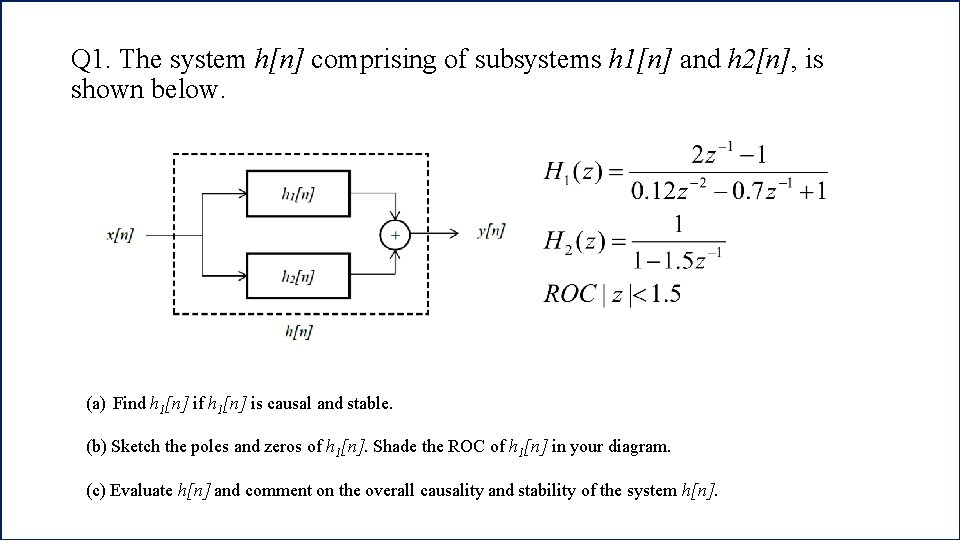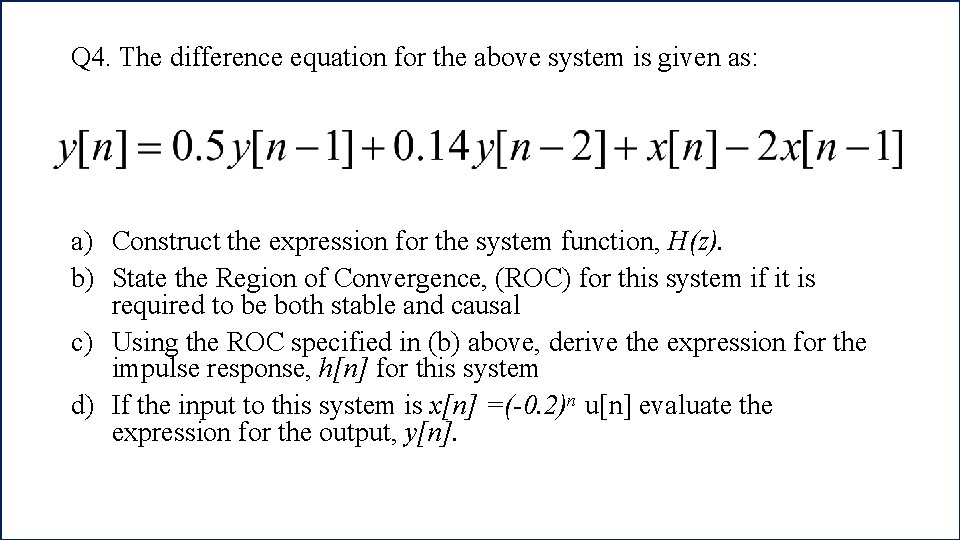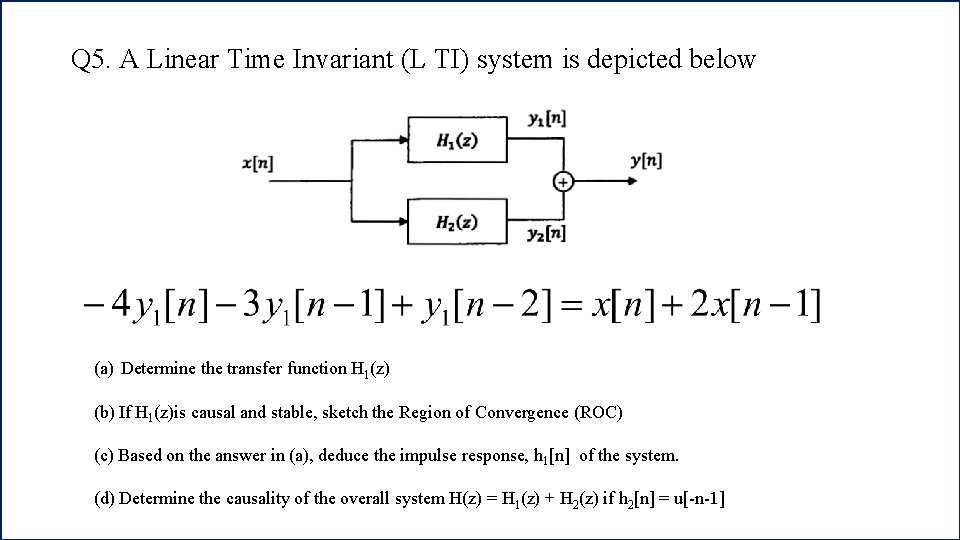# Chapter 5 Exercise Q 1 The system hn

• Slides: 6Chapter 5 ExerciseQ 1. The system h[n] comprising of subsystems h 1[n] and h 2[n], is shown below. (a) Find h 1[n] if h 1[n] is causal and stable. (b) Sketch the poles and zeros of h 1[n]. Shade the ROC of h 1[n] in your diagram. (c) Evaluate h[n] and comment on the overall causality and stability of the system h[n].Q 2. A digital filter is described by the following difference equation: a) Find the transfer function, H(z) of the digital filter b) Compute the impulse response, h(n) of the filter c) Sketch the poles and zeros of the filter and discuss its stabilityQ 3. Determine z-transform for the following problem. Sketch the polezero plot and indicate the region on convergence (ROC)Q 4. The difference equation for the above system is given as: a) Construct the expression for the system function, H(z). b) State the Region of Convergence, (ROC) for this system if it is required to be both stable and causal c) Using the ROC specified in (b) above, derive the expression for the impulse response, h[n] for this system d) If the input to this system is x[n] =(-0. 2)n u[n] evaluate the expression for the output, y[n].Q 5. A Linear Time Invariant (L TI) system is depicted below (a) Determine the transfer function H 1(z) (b) If H 1(z)is causal and stable, sketch the Region of Convergence (ROC) (c) Based on the answer in (a), deduce the impulse response, h 1[n] of the system. (d) Determine the causality of the overall system H(z) = H 1(z) + H 2(z) if h 2[n] = u[-n-1]